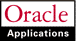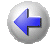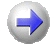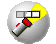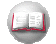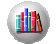Previous Next Contents Index Glossary Library

## Defining Unit of Measure Conversions

Unit of measure conversions are numerical factors that enable you to perform transactions in units other than the primary unit of the item being transacted. You can define:

Unit of measure conversions are not organization-specific.

You must define a conversion between a non-base unit of measure and the base unit of measure before you can assign the non-base unit of measure to an item.

#### Specifying Which Conversion to Use

When you define an item you decide which type of unit of measure conversion to use:

• Item specific: Only uses unit of measure conversions unique to this item. If none exist, you can only transact this item in its primary unit of measure.
• Standard: Uses standard unit of measure conversions for this item if an item-specific conversion is not available.
• Both: Uses both item-specific and standard unit of measure conversions. If both exist for the same unit of measure and item combination, the item-specific conversion is used.

#### Unit of Measure Conversions During Transactions

Whenever you enter an item's quantity, the default is the primary unit of measure for the item. The list of values for the unit of measure field displays all units of measure for which you have defined standard and/or item-specific conversions from the primary unit of measure.

Transactions are performed in the unit of measure you specify. The conversion happens automatically and item quantities are updated in the primary unit of measure of the item.

####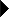To define a Standard conversion for any item:

2. Select the Standard alternative region.
3. Enter a unit of measure.
For example, if one DZ (this unit of measure) is equivalent to 12 EA (base unit), the conversion factor is 12. Or, if EA is equal to one-twelfth of a DZ, the conversion factor is 0.08333.

####To define a conversion for a specific item within a unit of measure class (Intra-class):

1. Navigate to the Unit of Measure Conversions window.
2. Select the Intra-class alternative region.
3. Enter an item.
4. Enter a unit of measure.
For example, if one LB (this unit of measure) is equivalent to 16 OZ (base unit), the conversion factor is 16.

####To define a conversion for a specific item between unit of measure classes (Inter-class):

1. Navigate to the Unit of Measure Conversions window.
2. Select the Inter-class alternative region.
3. Select an item.
4. Select the destination base unit of measure of the class to which you are converting a unit of measure.
For example, if one ML (source base unit) is equivalent to one GR (destination base unit), the conversion factor is one.

####To make a unit of measure conversion inactive:

• Enter the date on which the conversion becomes inactive.
As of this date, you can no longer use the unit of measure conversion.